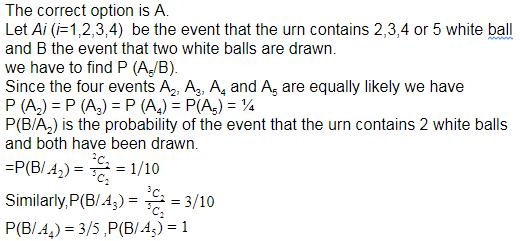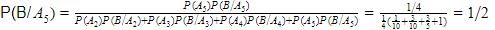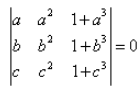# Test: Bayes’ Theorem

## 10 Questions MCQ Test Mathematics (Maths) Class 12 | Test: Bayes’ Theorem

Description
This mock test of Test: Bayes’ Theorem for JEE helps you for every JEE entrance exam. This contains 10 Multiple Choice Questions for JEE Test: Bayes’ Theorem (mcq) to study with solutions a complete question bank. The solved questions answers in this Test: Bayes’ Theorem quiz give you a good mix of easy questions and tough questions. JEE students definitely take this Test: Bayes’ Theorem exercise for a better result in the exam. You can find other Test: Bayes’ Theorem extra questions, long questions & short questions for JEE on EduRev as well by searching above.
QUESTION: 1

### An urn contains five balls. Two balls are drawn and found to be white. The probability that all the balls are white is:​

Solution:QUESTION: 2

### A bag ‘A’ contains 2 white and 3 red balls and a bag ‘B’ contains 4 white and 5 red balls. One ball is drawn at random from one of the bags and is found to be red. The probability that it was drawn from bag B is:​

Solution:

If we assume that it is equally likely that the ball is drawn from either bag, then we proceed as follows. Let R be the event that a red ball is drawn, and let B be the event that bag B is chosen for the drawing. Then
P(B) = P(not B) = 1/2
P(R|B) = 5/9 = P(RB) / P(B) = 2 P(RB),
P(R|not B) = 3/5 = P(R not B) / P(not B) = 2 P(R not B)
P(R) = P(RB) + P(R not B) = (1/2)( 5/9 + 3/5 ) = 26/45
P(B|R) = P(RB) / P(R) = (5/9)(1/2) / (26/45) = 25/52

QUESTION: 3

### If A and B are two events such that and P(B) ≠ 0, then which of the following is correct?

Solution:
QUESTION: 4

A doctor is to visit a patient. From the past experience, it is known that the probabilities that he will come by train, bus, and scooter or by other means of transport are respectively 0.3, 0.2, 0.1 and 0.4. The probabilities that he will be late are 1/4 , 1/3 and 1/12, if he comes by train, bus and scooter respectively, but if he comes by other means of transport, then he will not be late. When he arrives, he is late. The probability that he comes by bus is:​

Solution:

Let E1,E2,E3andE4 be the events that the doctor comes by train, bus, scooter and car respectively. Then,
P(E1)=3/10,P(E2)=1/5,P(E3)=1/10andP(E4)=2/5.
Let E be the event that the doctor is late. Then,
P(E/E1) = probability that the doctor is late, given that he comes by train
=1/4.
P(E/E2)= probability that the doctor is late, given that he comes by bus
=1/3.
P(E/E3)= probability that the doctor is late, given that the comes by scooter
=1/12.
P(E/E4)= probability that the doctor is late, given that that he comes by car
=0.
Probability that he comes by train, given that he is late
P(E2/E)
[P(E2).P(E/E2)]/[P(E1).P(E/E1)+P(E2).P(E/E2)+P(E3).P(E/E3)+P(E4).P(E/E40)][by Bayes's theorem]
[(1/5×1/3)]/(3/10×1/4)+(1/5×1/3)+(1/10×1/12)+(2/5×0)
=(3/40×120/18)=4/9
Hence, the required probability is 4/9.

QUESTION: 5

The conditional probability P(Ei |A) is called a ……… probability of the hypothesis Ei.​

Solution:
QUESTION: 6

Let {E1, E2, …, En} be a partition of the sample space S, and suppose that each of the events E1, E2,…, En has nonzero probability of occurrence. Let A be any event associated with S, then

Solution:
QUESTION: 7

If a, b and c are all distinct andthe

Solution:
QUESTION: 8

Probability that A speaks truth is 5/9 . A coin is tossed and reports that a head appears. The probability that actually there was head is:​

Solution:

Let E : A speaks truth
F : A lies
H : head appears on the toss of coin
P(E) = Probability A speaks truth
= 5/9
P(F) = Probability A speaks lies
1 - P(E) => 1-(5/9)
=> 4/9
P(H/E) = Probability that appears head, if A speaks truth  = ½
P(E/H) = Probability that appears head, if A speaks lies  = 1/2
P(H/E) = [(5/9) (½)]/[(5/9) (½) + (4/9) (½)]
= (5/18)/[(5/18) + (4/18)]
= 5/9
Let E : A speaks truth
F : A lies
H : head appears on the toss of coin
P(E) = Probability A speaks truth
= 5/9
P(F) = Probability A speaks lies
1 - P(E) => 1-(5/9)
=> 4/9
P(H/E) = Probability that appears head, if A speaks truth  = ½
P(E/H) = Probability that appears head, if A speaks lies  = 1/2
P(H/E) = [(5/9) (½)]/[(5/9) (½) + (4/9) (½)]
= (5/18)/[(5/18) + (4/18)]
= 5/9

QUESTION: 9

Bayes theorem is applicable when the events are:​

Solution:
QUESTION: 10

A man is known to speak truth 3 out of 4 times. He throws a die and reports that it is a six. Find the probability that it is actually a six.​

Solution:

Let E be the event that the man reports that six occurs in the throwing of the die and
let S1 be the event that six occurs and S2 be the event that six does not occur.  Then P(S1) = Probability that six occurs = 1/6
P(S2) = Probability that six does not occur = 5/6
P(E|S1) = Probability that the man reports that six occurs when six has actually occurred on the die
= Probability that the man speaks the truth = 3/4
P(E|S2) = Probability that the man reports that six occurs when six has not actually occurred on the die
= Probability that the man does not speak the truth = 1 - 3/4 = 1/4
Thus, by Bayes' theorem, we get
P(S1|E) = Probability that the report of the man that six has occurred is actually a six
⇒P(S1/E) = (P(S1)P(E/S1))/(P(S1)P(E/S1) + P(S2)P(E/S2))
= (1/6 x 3/4)/(1/6 x 3/4 + 5/6 x 1/4)
= 1/8 x 24/8
= 3/8